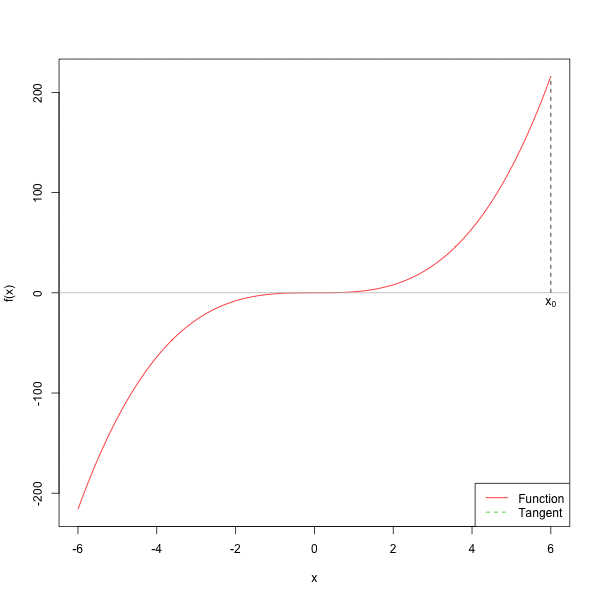# 第 2 章 求根算法

## 2.2 牛顿法

$0 = f(x^*) = f(x_0+h) \approx f(x_0) + h f^{\prime}(x_0),$

$h \approx-\frac{f\left(x_{0}\right)}{f^{\prime}\left(x_{0}\right)}.$

$x^*=x_{0}+h \approx x_{0}-\frac{f\left(x_{0}\right)}{f^{\prime}\left(x_{0}\right)},$

$x_{1}=x_{0}-\frac{f\left(x_{0}\right)}{f^{\prime}\left(x_{0}\right)}.$

$x_{2}=x_{1}-\frac{f\left(x_{1}\right)}{f^{\prime}\left(x_{1}\right)}.$

1. 选择初始猜测点 $$x_0$$，设置 $$n = 0$$

2. 按照以下迭代过程进行迭代：

$\begin{equation} x_{n+1}=x_{n}-\frac{f\left(x_{n}\right)}{f^{\prime}\left(x_{n}\right)}. \end{equation}$

1. 计算 $$|f(x_{n+1})|$$

1. 如果 $$|f(x_{n+1})| \leq \epsilon$$，停止迭代；
2. 否则，返回第 2 步。newton.root <- function(f, x0 = 0, tol = 1e-9,
max.iter = 100) {
x <- x0
cat(paste0("初始值：x = ", x, "\n"))
fx <- ftn(f, x)
iter <-  0
# xs用来保存每步迭代得到的x值
xs <- list()
xs[[iter + 1]] <- x
# 继续迭代直到满足停止条件
while ((abs(fx$f) > tol) && (iter < max.iter)) { x <- x - fx$f/fx$fgrad fx <- ftn(f, x) iter <- iter + 1 xs[[iter + 1]] <- x cat(paste0("迭代第", iter, "次：x = ", x, "\n")) } # output depends on success of algorithm if (abs(fx$f) > tol) {
cat("算法无法收敛\n")
return(NULL)
} else {
cat("算法收敛\n")
return(xs)
}
}

ftn <- function(f, x){
df <- deriv(f, 'x', func = TRUE)
dfx <- df(x)
f <- dfx
}
f <- expression(x^2 - 5)
#> 算法收敛